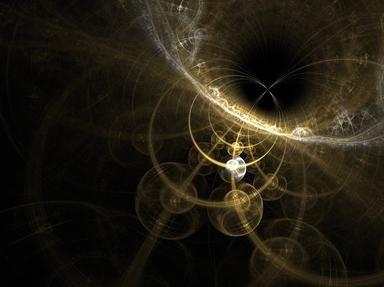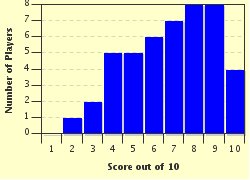FREE! Click here to Join FunTrivia. Thousands of games, quizzes, and lots more!# Another Quiz on Quantum Mechanics

### Quantum mechanics has provided scientists with some of the most puzzling phenomena known to mankind. Feel free to test your knowledge of this tricky subject in this quiz.

A multiple-choice quiz by happy1234512. Estimated time: 4 mins.

Author
Time
4 mins
Type
Multiple Choice
Quiz #
388,695
Updated
Jun 03 22
# Qns
10
Difficulty
Average
Avg Score
7 / 10
Plays
413
Awards
Top 35% Quiz
- -
Question 1 of 10
1. Which of the four fundamental forces binds quarks together to form atomic nuclei? Hint

#### NEXT>

Question 2 of 10
2. The ultraviolet catastrophe was a term given to the failure of classical physics to predict the emission spectrum of a blackbody.

#### NEXT>

Question 3 of 10
3. Why is Bohr's planetary model of the atom inaccurate? Hint

#### NEXT>

Question 4 of 10
4. What is the name given to the constant that relates the energy of a photon to its frequency? Hint

#### NEXT>

Question 5 of 10
5. Angular momentum is a scalar quantity.

#### NEXT>

Question 6 of 10
6. Which universal constant can be calculated by multiplying a photon's frequency by its wavelength? Hint

#### NEXT>

Question 7 of 10
7. Which of the following types of waves requires a medium to travel through? Hint

#### NEXT>

Question 8 of 10
8. According to Einstein's theory of general relativity, gravity can be defined as a distortion in space-time.

#### NEXT>

Question 9 of 10
9. Which of the following particles is classified as a force-carrying particle? Hint

#### NEXT>

Question 10 of 10
10. Which of the following scientists was never directly involved in the field of quantum mechanics? Hint

 (Optional) Create a Free FunTrivia ID to save the points you are about to earn:Select a User ID:Choose a Password:Your Email:

Most Recent Scores
Nov 24 2023 : thok: 8/10
Nov 24 2023 : Guest 146: 3/10

Score DistributionQuiz Answer Key and Fun Facts
1. Which of the four fundamental forces binds quarks together to form atomic nuclei?

The strong interaction is responsible for holding together quarks to form larger, more composite particles. Strong interaction is commonly known to be present in atomic nuclei, where it holds together neutrons, protons, and the overall nucleus. Neutrons contain two down quarks and one up quark while protons contain two up quarks and one down quark.

These hadrons are bound together by gluons, the force-carrying particles of the strong interaction.
2. The ultraviolet catastrophe was a term given to the failure of classical physics to predict the emission spectrum of a blackbody.

A blackbody is a theoretical object that absorbs all radiation on its surface and perfectly remits it all. According to classical physics, higher thermal energy would cause the blackbody to radiate lower wavelength radiation. While this was true, classical physics also predicted that as the wavelength of the radiated energy decreased, the blackbody would eventually emit infinite amounts of energy.

This theory was quite far from the experimental data, which showed that the energy would only increase until it reached the ultraviolet region of the EM spectrum, and would then plummet down. On a graph where energy was plotted against wavelength, the famous blackbody radiation curve was formed. Physicists referred to this error in classical physics as "the ultraviolet catastrophe".
3. Why is Bohr's planetary model of the atom inaccurate?

Answer: because it implies that we can figure out the exact orbital path of an electron

Niels Bohr(1885-1962) was a Danish scientist who proposed the planetary model of the atom. This model was correct in some aspects, as it accurately showed the neutrons and protons concentrated in the center of the atom, whilst the electrons orbited around them.

However, the reason that this model isn't completely accurate is because it implies that electrons follow orbits much like those of the planets - ones that we can accurately measure. According to Heisenberg's Uncertainty Principle, it is impossible to simultaneously know the exact position and momentum of a particle.

Therefore, it is not possible to measure the exact orbital path of an electron. More modern models of the atom show that it is only possible to measure the probability of finding an electron in a specific place at a specific time. Orbitals refer to these specific areas in an atom where there is a high probability of finding any specific electron.
4. What is the name given to the constant that relates the energy of a photon to its frequency?

Planck's constant is named after Max Planck (1858-1947), a German physicist. Planck is best known for providing a mathematical solution to the ultraviolet catastrophe, .and thus discovering quantum mechanics. Contrary to classical mechanics, Planck theorized that energy is quantized.

This meant that energy could only be absorbed and emitted in discrete packets, which we now call photons. Plank derived the equation E=hv, where E represents energy, v represents frequency, and h represents a constant which he named after himself.

It is equal to approximately 6.626 x 10^-34 Joule-seconds.
5. Angular momentum is a scalar quantity.

Angular momentum is a measure of a body's inclination to keep rotating. This quantity can be derived by multiplying the body's moment of inertia by its angular velocity. Angular momentum can be described in terms of both magnitude and direction, thus making it a vector quantity. Electrons possess two types of angular momentum: orbital angular momentum and intrinsic angular momentum.

The magnitude of an electron's orbital angular momentum can be described by its angular momentum quantum number, while its intrinsic angular momentum can be described by its spin quantum number.
6. Which universal constant can be calculated by multiplying a photon's frequency by its wavelength?

The speed of light is equal to about 300,000,000 meters per second. At a constant speed, a wave's wavelength is inversely proportional to its frequency. Photons are the force carrying particle of the electromagnetic force, and therefore travel at the speed of light. Thus, the product of the photon's frequency and wavelength is always equal to this universal constant.
7. Which of the following types of waves requires a medium to travel through?

Mechanical waves always require a medium to travel through due to the fact that they occur from the vibration of the particles that are part of the medium. Sound is a mechanical wave and thus requires a medium to travel through. On earth, air commonly acts as a medium to travel through.

However, sound can also travel through liquids and solids. Ultraviolet waves, microwaves, and radio waves are all electromagnetic waves, and can therefore travel through a vacuum.
8. According to Einstein's theory of general relativity, gravity can be defined as a distortion in space-time.

Einstein's general theory of relativity introduced the concept of space-time. According to this theory, massive objects warp space-time, causing it to curve in specific areas. For example, Newton's law of gravity tells us that earth orbits the sun, and therefore accelerates, due to the sun's gravitational pull.

However, Einstein's general theory of relativity states that the curve of space time caused by the sun's mass causes the earth to accelerate and thus orbit the sun. Therefore, Newton's definition of the force of gravity would merely be a distortion in space time.
9. Which of the following particles is classified as a force-carrying particle?

Bosons are classified as force-carrying particles. The W boson along with the z boson are the carriers of the weak force, which occurs during nuclear decay. Photons, gluons, and gravitons are also bosons. Photons carry the electromagnetic force, gluons carry the strong force, and gravitons carry the gravitational force. Electrons, positrons, and neutrinos are all classified as leptons, meaning they constitute matter.
10. Which of the following scientists was never directly involved in the field of quantum mechanics?